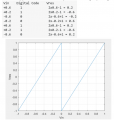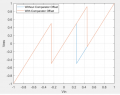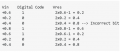# why changing reference range fixes offset error

#### yef smith

Joined Aug 2, 2020
112
Hello,bellow we have two pipeline adc stages,
the first is 7 bit from 1 bit stage ,the other is 7 bit from 1.5 bit stages.
For the first pipe line shown bellow with input 0.6v our voltage input ranges from -1 to 1 , In decimal system 1100110 is 102 which corresponds to 102/128 -1 =0.59V .For second pipeline shown bellow with 1.5bit pipeline ADC with one of the comparators having 0.21V offset error as shown in the diagram bellowIf 0.6V input is applied to this ADC, the output code is 1001100 with the calculations shown bellow in decimal its 76.
and if we convert this 76 by a range of 0-1 then (76/128)*1=0.59 thus we get the correct result when we chane the Vin range .

But in 1.5 its from -1 to 1 too, its not 0 to 1,why in the final result we are changing the reference input range ,and what is the logic behind it to solve offset errors in pipeline?
Thanks.Last edited: